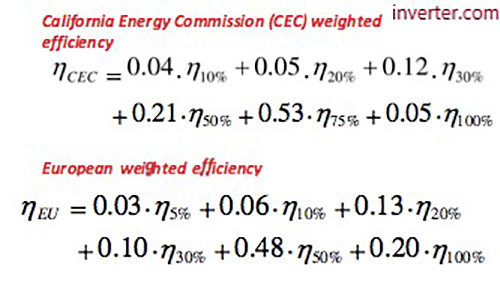# What is Inverter Efficiency?

What does inverter efficiency mean?

In fact, we shall discuss here the general power inverter efficiency whether it’s solar inverter or pure sine wave inverter or even modified sine wave inverter.

The inverter efficiency refers to how much dc power will be converted to ac power, as some of power will be lost during this transition in two forms:

• Heat loss.
• Stand-by power which consumed just to keep the inverter in power mode. Also, we can refer to it as inverter power consumption at no load condition.

Hence, inverter efficiency = pac/pdc where pac refers to ac output power in watt and pdc refers to dc input power in watts.

For the two basic inverters types in the market, the typical efficiency of high-quality pure sine wave inverter varied from 90% to 95% and for low quality modified sine wave inverter, it varied from 75% to 85%.

This power inverter efficiency value depends on inverter load power capacity variation, as the efficiency increases and may reach to its max value at higher load power capacity in compare to lower loading power capacity, and in condition that not going above inverter output power capacity limit. Generally, below 15% inverter loading, the efficiency will be quite low. Consequently, good matching between inverter capacity and its load capacity will enable us harvest larger efficiency, which means larger inverter ac output power for the same dc input power.Inverter efficiency types:

There are three types of inverter efficiency, we should know the meaning of each one and the difference in between for better understanding of different inverters data sheet models and manufacturers in the market, as following:• Peak efficiency: It refers to max inverter efficiency value that can be reached at optimal output ac power, so it can be used just as an indication for the inverter quality.
• European efficiency: It refers to inverter efficiency measured at different ac output power points, then multiplied by different weighted number, so it’s more useful than peak efficiency because its shows how inverter performs at different output power during the solar day.
• California energy commission efficiency: It refers to inverter efficiency measured at different ac output power points, then multiplied by different weighted number, so it’s same like European efficiency, but it uses different multiplied weighted numbers (different calculation formula).

So, the main difference between the European and California efficiencies is the importance of each ac output power level when calculating efficiency for particular inverter.